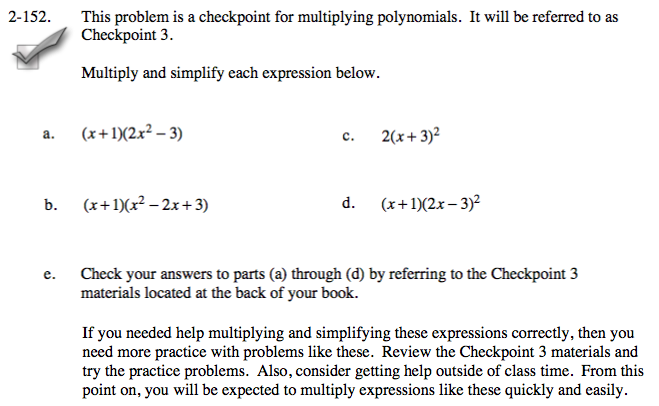### Home > CAAC > Chapter 13 > Lesson 13.RE3-S > Problem2-152

2-152.
1. This problem is a checkpoint for multiplying polynomials. It will be referred to as Checkpoint 3. Homework Help ✎Multiply and simplify each expression below.

1. (x + 1)(2x2 − 3)

2. (x + 1)(x2 − 2x + 3)

3. 2(x + 3)2

4. (x + 1)(2x − 3)2

5. Check your answers to parts (a) through (d) by referring to the Checkpoint 3 materials located at the back of your book.

If you needed help multiplying and simplifying these expressions correctly, then you need more practice with problems like these. Review the Checkpoint 3 materials and try the practice problems. Also, consider getting help outside of class time. From this point on, you will be expected to multiply expressions like these quickly and easily.Answers and extra practice are located in the back of your printed textbook or in the Reference Tab of your eBook.
If you have an eBook for A2C, login and then click the following link: Checkpoint 3 Answers and Practice